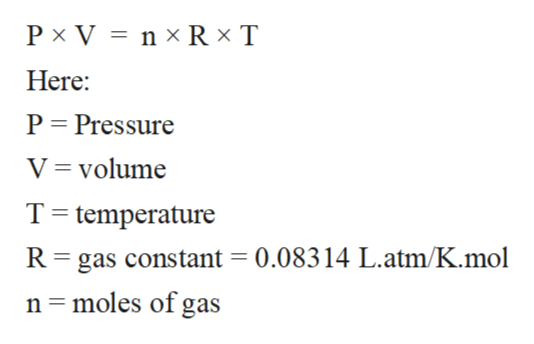# A balloon is filled with 66.7 g of H2 gas at STP. Calculate the volume of the H2 gas in liters. Refer to the periodic table as needed.

Question
14 views

A balloon is filled with 66.7 g of H2 gas at STP. Calculate the volume of the H2 gas in liters. Refer to the periodic table as needed.

check_circle

Step 1

All matter can be classified as solid, liquid and gases mainly. In these physical states of matter, the solid state is composed of particles with least intermolecular distance and strongest intermolecular force of attraction. On the contrary, the gaseous state of matter is composed of particles with largest intermolecular distance and weakest intermolecular force of attraction. The liquid state of matter exhibits the intermediate properties of them.

Step 2

The gaseous state is the state of matter with least intermolecular force of attraction between molecules and highest kinetic energy. The standard properties of gases like Pressure, volume, and temperature can be calculated with the help of ideal gas equation.

The ideal gas equation can be written as:help_outlineImage TranscriptioncloseP x V nx R x T Here: P Pressure V volume T temperature R gas constant = 0.08314 L.atm/K.mol n moles of gas fullscreen
Step 3

At STP conditions;

1 mole of ideal gas = 22.4 L of gas

Calculate the volume of gas:

Mass o...

### Want to see the full answer?

See Solution

#### Want to see this answer and more?

Solutions are written by subject experts who are available 24/7. Questions are typically answered within 1 hour.*

See Solution
*Response times may vary by subject and question.
Tagged in

### Ideal gases